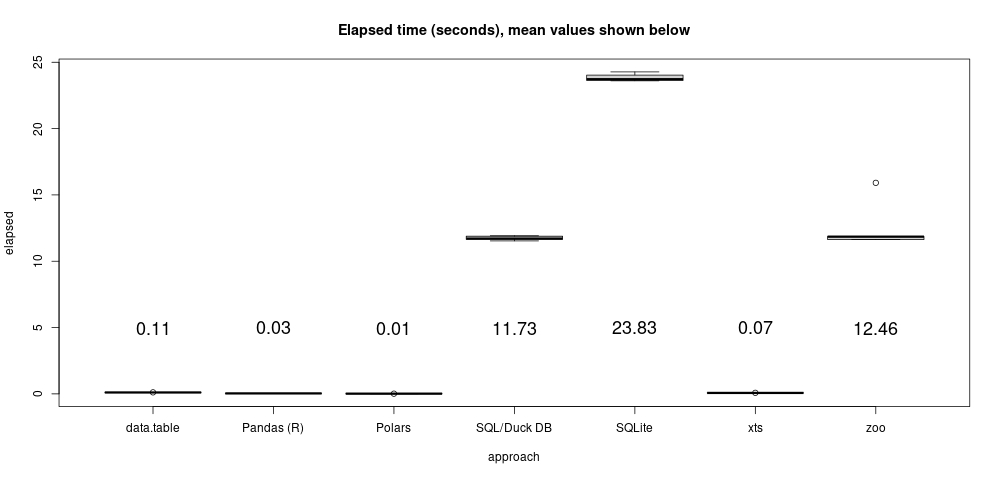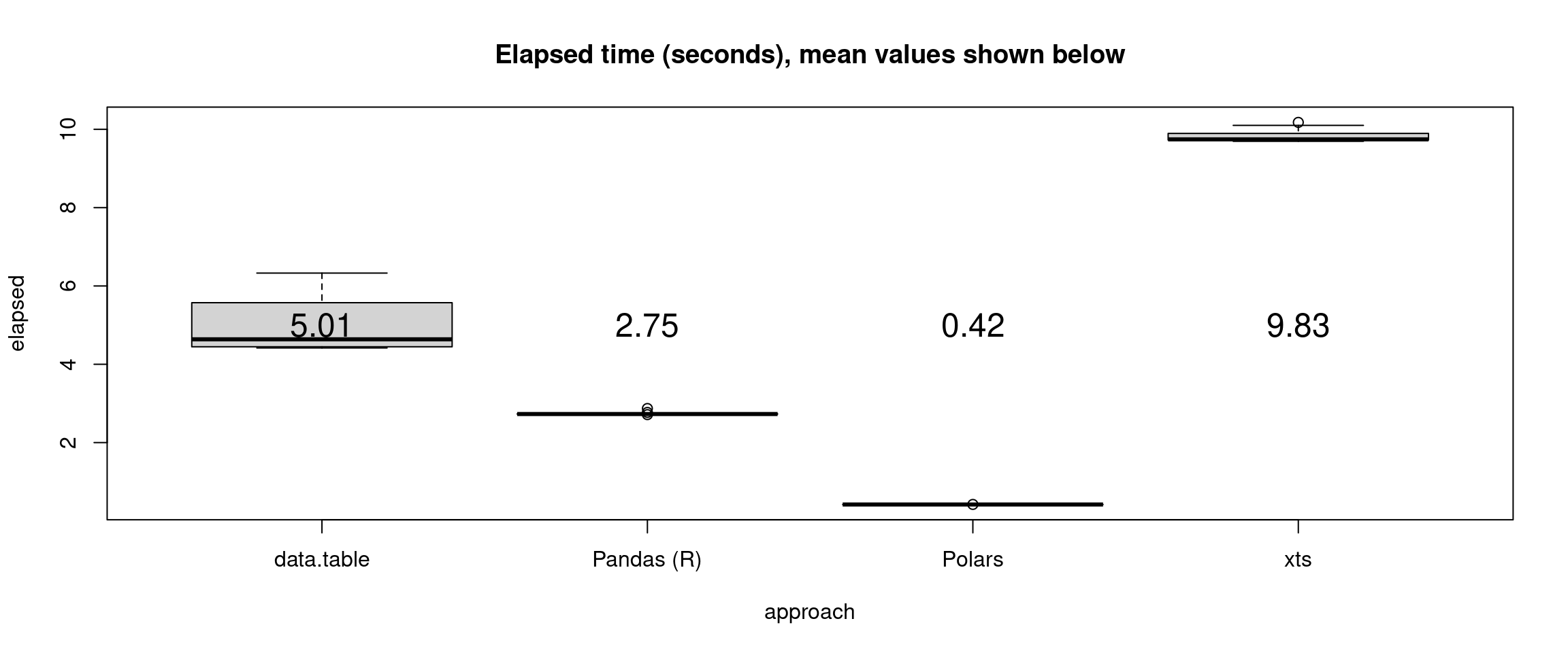# “As of”-style joins

This piece describes a kind of table join common in time series problems (remote sensing, IOT, finance, telemetry logging, communications, etc.). It proceeds in two parts: first, a very simple example and some solutions to illustrate key ideas; then a larger example to illustrate performance.

I’ve updated this post on March 2022 to include results from Polars and the latest versions of DuckDB (which continues to improve significantly).

## Part 1: The basic idea

Given a table of desired dates, or times, or indeed any ordered values (call this table “calendar”), and a table of dates and data values (call this table “data”), the gist is to produce an output table with dates corresponding to the “calendar” table and values corresponding to the most recently known value from the “data” table. In other words, find the last known value as of each calendar date (thus the title).

Consider the example “calendar” and “data” tables, along with the desired “as of” join output table shown below. Note, in particular, that not all dates in the “calendar” table are present in the “data” table.

calendar
date
2020-01-01
2020-02-01
2020-03-01
2020-04-01
2020-05-01
2020-06-01
data
date value
2019-11-18 0.6870228
2020-01-05 0.0617863
2020-01-10 0.1765568
2020-02-01 0.5000000
2020-02-12 0.2059746
2020-04-13 0.6291140
2020-05-08 0.3841037
‘as of’ desired output
date value
2020-01-01 0.6870228
2020-02-01 0.5000000
2020-03-01 0.2059746
2020-04-01 0.2059746
2020-05-01 0.6291140
2020-06-01 0.3841037

You can think about the “as of” join in different ways:

1. As a full outer join between the tables on date, followed by piece-wise constant interpolation of any missing values, followed by an inner join on date with the “calendar” table.
2. As a kind of inequality rolling join, where, for each “calendar” table date, join the value associated with the last “data” table date less than or equal to the “calendar” table date.
3. Probably many other ways…

This note illustrates both approaches 1 and 2.

Because “as of” style joins are so common in time series settings, most time series-specific databases usually handle this kind of thing pretty easily–for instance, the commercial Kdb+ database handles such joins with simple, concise syntax and extreme performance.

R and Python, and now Rust (Polars), are very good for time series problems and also exhibit several ways to carry out “as of” style joins (with varying levels of performance).

ANSI SQL is probably not the best way to express solutions to the “as of” join problem (as we shall see), but it can be done. However, many SQL databases include idiosyncratic approaches to more efficiently deal with this. Every SQL approach I have seen tends to be (to me, at least) over-complicated (and slow).

If you don’t feel like reading any more, the quick take away is, use Polars, Python Pandas, R xts, data.table, or Kdb+ for this kind of problem if you need performance.

### Some R approaches

Let’s explore two possible R approaches to formulating a solution. The first zoo-package approach conceptually follows way 1 above (piecewise constant interpolation of missing values after an outer join). The second, data.table-package approach hews to way 2 above (a rolling non-equi join).

First set up the simple example ‘calendar’ and ‘data’ data.frames in R:

set.seed(1)
start <- as.Date("2020-06-20")
calendar <- data.frame(date = seq(from = as.Date(format(start, "%Y-%m-01")), length.out = 6, by = "-1 month"))
calendar <- calendar[order(calendar[["date"]]),, drop=FALSE]

data <- data.frame(date = start - sample(240, 6, replace = TRUE), value = runif(6))
data <- rbind(data,  cbind(calendar[2,,drop = FALSE], value = 0.5))
data <- data[order(data[["date"]]),]

These data.frames look just like the example tables shown above. Now, a zoo approach. It uses zoo’s na.locf function, an acronym for “missing value, last observation carry-forward.”

library(zoo)
(ans.zoo <- merge(calendar, na.locf(merge(calendar, data, all = TRUE)), all.x = TRUE))
##         date     value
## 1 2020-01-01 0.6870228
## 2 2020-02-01 0.5000000
## 3 2020-03-01 0.2059746
## 4 2020-04-01 0.2059746
## 5 2020-05-01 0.6291140
## 6 2020-06-01 0.3841037

R’s xts package can work in the same way as the zoo approach above, but much much faster. That will be illustrated in the performance section below.

Data.table’s “rolling join” approach produces the same result with a nicely concise syntax:

library(data.table)
data.dt <- data.table(data)
(ans.dt <- data.dt[calendar, on = "date", roll = TRUE])
##          date     value
## 1: 2020-01-01 0.6870228
## 2: 2020-02-01 0.5000000
## 3: 2020-03-01 0.2059746
## 4: 2020-04-01 0.2059746
## 5: 2020-05-01 0.6291140
## 6: 2020-06-01 0.3841037

The rolling data.table join is quite flexible and allows for limiting the extent of the search for a last value, among several other options. Also note that both approaches also work in cases when the “data” table has more than one column (possibly each with missing values).

### A Python Pandas approach

Pandas includes a merge_asof method that is very similar to data.table’s rolling joins. It doesn’t seem to work directly with R’s Date type though, so we need to convert the date columns to full date-time POSIXct first:

calendar.posix <- data.frame(date = as.POSIXct(calendar[["date"]]))
data.posix <- data.frame(date = as.POSIXct(data[["date"]]), value = data[["value"]])

library(reticulate)
pandas <- import("pandas")
ans.py <- pandas$merge_asof(calendar.posix, data.posix, on = "date") ans.py[["date"]] <- as.Date(ans.py[["date"]]) ans.py ## date value ## 0 2020-01-01 0.6870228 ## 1 2020-02-01 0.5000000 ## 2 2020-03-01 0.2059746 ## 3 2020-04-01 0.2059746 ## 4 2020-05-01 0.6291140 ## 5 2020-06-01 0.3841037 ### SQL It took me a while to come up with a generic SQL approach (try it yourself!). Internet searches for phrases like “SQL as.of-style join,” “SQL last value fill in join,” and so on return many results of variable quality, most (all?) involving idiosyncratic syntax specific to a particular (often commercial) database (for example Microsoft SQL Server, or Oracle databases, etc.). Giving up on the internet, I was at least able to cook up the following working vanilla SQL example. Reader, be advised: the following material may offend your sensibilities! library(duckdb) con <- dbConnect(duckdb()) duckdb_register(con, "data", data) duckdb_register(con, "calendar", calendar) Q <- "WITH z AS ( SELECT date, (NULL) AS value FROM calendar UNION SELECT date, value FROM data ORDER BY date ), a AS ( SELECT date, value, ROW_NUMBER() OVER ( ORDER BY date RANGE BETWEEN UNBOUNDED PRECEDING AND CURRENT ROW ) * (CASE WHEN value IS NULL THEN 0 ELSE 1 END) AS i FROM z ), b AS ( SELECT date, MAX(i) OVER ( ORDER BY date RANGE BETWEEN UNBOUNDED PRECEDING AND CURRENT ROW ) AS j FROM a ), c AS ( SELECT b.date, value FROM a, b WHERE a.i > 0 AND a.i = b.j ), d AS ( SELECT calendar.date, value FROM calendar, c WHERE calendar.date = c.date ORDER BY c.date ) SELECT * FROM d UNION SELECT * FROM d " (ans.duck <- dbGetQuery(con, Q)) ## date value ## 1 2020-01-01 0.6870228 ## 2 2020-02-01 0.5000000 ## 3 2020-03-01 0.2059746 ## 4 2020-04-01 0.2059746 ## 5 2020-05-01 0.6291140 ## 6 2020-06-01 0.3841037 The generic SQL approach syntax is horrible. I guess that’s part of the point of all of these examples, sometimes SQL is just not the right tool for the job. Of course, I am far from an expert–if you can find a better way to formulate a solution to this problem in plain vanilla SQL let me know (send a pull request or whatever)! ## Part 2: Performance Keeping things simple, performance is explored below by running a bigger version of the example from Part 1 above. Except, it’s not really all that big, 5 million “data” table observations and about 250 thousand (every minute) calendar table desired date/times. This example uses POSIXct date/time values instead of simple R Date (date only) values used in the example above. It easily fits into the main memory of my 8GB laptop, but is sufficiently large to start to see performance differences between approaches. Here is the data setup: set.seed(1) end <- as.POSIXct("2020-06-20") start <- as.POSIXct("2020-1-1") dt <- as.integer(difftime(end, start, units = "secs")) # Every minute calendar <- data.frame(date = seq(from = start, to = end, by = "+1 min")) N <- 5e6 data <- data.frame(date = end - runif(N) * dt, value = runif(N)) data <- data[order(data[["date"]]),] Each approach proceeds as in Part 1, with two new approaches, summarized along with brief comments below. But first, the performance timing results:Xts and data.table are, as expected, very fast. This example is too small to really put those efficient R packages through their paces. Despite a conceptually identical approach to xts, the zoo way is quite a bit slower. Pandas and, especially, Polars are faster yet. I’m not surprised that the SQL solutions ran slowly, if for no other reason than the offensive query I wrote. ## A bigger problem R’s xts and data.table and Python Pandas and Polars are much faster than the other approaches. So much so that this problem is probably too small to test them well (set up overhead time is a large part of overall run time). We re-ran a larger problem simply by scaling up N <- 5e8 above. That’s two orders of magnitude larger than in the above tests. This problem exceeds the paltry 8 GB memory on my cheap laptop, so I ran on the following large system: one Amazon AWS Hpc6a.48xlarge instace with 96 physical AMD EPYC 7003 CPU cores and 384 GiB system RAM. The tested version of R was 4.1.3, Python 3.9.2, xts version 0.12.1, data.table 1.14.2, Pandas 1.1.5 and Polars 0.13.12 (via the Python interface).The Python packages exhibit very high performance, in particular Polars. Note that all of the illustrated R and Python approaches compute this as-of join faster than either SQL DB approach took to complete a problem two orders of magnitude smaller. ## Details Basic details for each approach are summarized below. ### Zoo library(zoo) t.zoo <- replicate(6, system.time({ ans.zoo <<- merge(calendar, na.locf(merge(calendar, data, all = TRUE)), all.x = TRUE) })) ### Xts R’s xts package defines a high-performance ordered index class that substantially expands on functions and ideas from the zoo package. It has many convenient functions and can solve this problem in more than one way. In particular, it can follow the same zoo approach used above (but runs much faster): library(xts) calendar.xts <- xts(, order.by = calendar[["date"]]) data.xts <- xts(data[["value"]], order.by = data[["date"]]) t.xts <- replicate(10, system.time({ ans.xts <<- merge(calendar.xts, na.locf(merge(calendar.xts, data.xts)), join = "left") })) ### Data.table The data.table approach is exactly as in Part 1 above: library(data.table) setDTthreads(8) data.dt <- data.table(data) t.dt <- replicate(10, system.time({ ans.dt <<- data.dt[calendar, on = "date", roll = TRUE] })) ### Python Pandas Because this example already uses POSIXct date/times, we don’t need to convert as in the simple example in Part 1. Otherwise identical. library(reticulate) pandas <- import("pandas", convert = FALSE) calendar_py <- r_to_py(calendar) data_py <- r_to_py(data) ans.py <- pandas$merge_asof(calendar_py, data_py, on = "date")
t.py <- replicate(10, system.time({